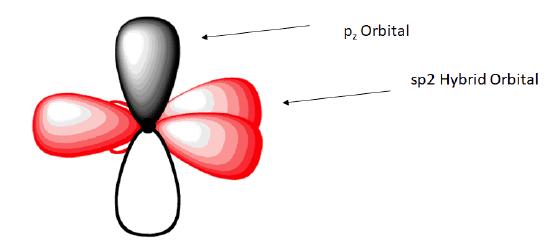# 8.2: Introduction to Alkenes

$$\newcommand{\vecs}{\overset { \rightharpoonup} {\mathbf{#1}} }$$ $$\newcommand{\vecd}{\overset{-\!-\!\rightharpoonup}{\vphantom{a}\smash {#1}}}$$$$\newcommand{\id}{\mathrm{id}}$$ $$\newcommand{\Span}{\mathrm{span}}$$ $$\newcommand{\kernel}{\mathrm{null}\,}$$ $$\newcommand{\range}{\mathrm{range}\,}$$ $$\newcommand{\RealPart}{\mathrm{Re}}$$ $$\newcommand{\ImaginaryPart}{\mathrm{Im}}$$ $$\newcommand{\Argument}{\mathrm{Arg}}$$ $$\newcommand{\norm}{\| #1 \|}$$ $$\newcommand{\inner}{\langle #1, #2 \rangle}$$ $$\newcommand{\Span}{\mathrm{span}}$$ $$\newcommand{\id}{\mathrm{id}}$$ $$\newcommand{\Span}{\mathrm{span}}$$ $$\newcommand{\kernel}{\mathrm{null}\,}$$ $$\newcommand{\range}{\mathrm{range}\,}$$ $$\newcommand{\RealPart}{\mathrm{Re}}$$ $$\newcommand{\ImaginaryPart}{\mathrm{Im}}$$ $$\newcommand{\Argument}{\mathrm{Arg}}$$ $$\newcommand{\norm}{\| #1 \|}$$ $$\newcommand{\inner}{\langle #1, #2 \rangle}$$ $$\newcommand{\Span}{\mathrm{span}}$$$$\newcommand{\AA}{\unicode[.8,0]{x212B}}$$

##### Objective

After completing this section, you should be able to give an example of a naturally occurring compound that contains at least one double bond.

##### Key Terms

Make certain that you can define, and use in context, the key term below.

• olefin
##### Study Notes

Alkenes are a class of hydrocarbons (i.e., containing only carbon and hydrogen). They are unsaturated compounds with at least one carbon-to-carbon double bond. The double bond makes Alkenes more reactive than alkanes. Olefin is another term used to describe alkenes.

The graphic shows three alkenes. The more complex alkene is commonly known as 1-menthene, but its full proper IUPAC name is 1-methyl-2-(1-methylethyl)-cyclohexene.## Alkenes

Alkenes are a class of hydrocarbons (i.e., containing only carbon and hydrogen). They are unsaturated compounds with at least one carbon-to-carbon double bond. The double bond makes alkenes more reactive than alkanes. Olefin is another term used to describe alkenes. The alkene group can also be called a vinyl group and the carbons sharing the double bond can be called vinyl carbons.## Structure of Ethene - the Simplest Alkene

Ethene is often written as CH2=CH2 which stands for:The double bond is shared by the two carbon atoms and does not involve the hydrogen atoms, although the condensed formula does not make this point obvious. Note that the molecular formula for ethene is C2H4, whereas that for ethane is C2H6.

Ethene is not a very complicated molecule. It is made up of four 1s1 hydrogen atoms and two 2s2 2px1 2py1 carbon atoms. These carbon atoms already have four electrons, but they each want to get four more so that they have a full eight (octet) in the valence shell. Having eight valence electrons around carbon gives the atom the same electron configuration as neon, a noble gas. Carbon wants to have the same configuration as neon because when it has eight valence electrons carbon is at its most stable, lowest energy state.This forms a total of three bonds to each carbon atom, giving them an $$sp^2$$ hybridization. Since the carbon atom is forming three sigma bonds instead of the four that it can, it only needs to hybridize three of its outer orbitals, instead of four. It does this by using the $$2s$$ electron and two of the $$2p$$ electrons, leaving the other unchanged. This new orbital is called an $$sp^2$$ hybrid because it is made from one s orbital and two p orbitals.When atoms use $$sp^2$$ hybrids they have a trigonal planar structure. These structures are very similar to a 'peace' sign, there is a central atom with three atoms around it, all on one plane. Trigonal planar molecules have an ideal bond angle of 120° on each side.The H-C-H bond angle is 117°, which is very close to the ideal 120° of a carbon with $$sp^2$$ hybridization. The other two angles (H-C=C) are both 121.5°.

### Rigidity in Ethene

There is rigidity in the ethene molecule due to the double-bonded carbons. A double bond consists of one sigma bond formed by overlap of sp2 hybrid orbitals and one pi bond formed by overlap of parallel 2 p orbitals. In ethene there is no free rotation about the carbon-carbon sigma bond because these two carbons also share a $$\pi$$ bond. A $$\pi$$ bond is only formed when there is adequate overlap between both top and bottom p-orbitals. Rotation of the p-orbitals causes them to be 90° from each other breaking the $$\pi$$ bond because there would be no overlap. There is a much larger energy barrier to rotation than there is about a carbon-carbon sigma bond.(a) The σ-bonded framework is formed by the overlap of two sets of singly occupied carbon sp2 hybrid orbitals and four singly occupied hydrogen 1s orbitals to form electron-pair bonds. This uses 10 of the 12 valence electrons to form a total of five σ bonds (four C–H bonds and one C–C bond).

(b) One singly occupied unhybridized 2pz orbital remains on each carbon atom to form a carbon–carbon π bond. (Note: by convention, in planar molecules the axis perpendicular to the molecular plane is the z-axis.)

##### Exercise

1. Although there is only one alkene with the formula C2H4 (ethene) and only one with the formula C3H6 (propene), there are several alkenes with the formula C4H8. Draw all of the possible bond line structures for alkenes with the formula C4H8 including all possible structural and stereoisomers.